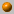##### Personal tools
You are here: Home Computational Geometry - Fall 2016/2017
« February 2023 »
February
SuMoTuWeThFrSa
1234
567891011
12131415161718
19202122232425
262728

# Computational Geometry - Fall 2016/2017

Monday, 16:00-19:00, Schreiber 006

Instructor: Dan Halperin , danha@post
Office hours: by appointment

TA: Nitzan Weisman, nitzanw@mail.tau.ac.il
Office hours: by appointment

Grader: Shahar Shamai, shasha94 AT gmail.com
Maibox no. 312, Schriebr, 1st floor

The course covers fundamental algorithms for solving geometric problems such as computing convex hulls, intersection of line segments, Voronoi diagrams, polygon triangulation, and linear programming in low dimensional space. We will also discuss several applications of geometric algorithms to solving problems in robotics, GIS (geographic information systems), computer graphics, and more.

### Bibliography:

The main textbook of the course is:

Computational Geometry: Algorithms and Applications (CGAA), 2nd or 3rd edition by M. de Berg, M. van Kreveld, M. Overmars, and O. Schwarzkopf.

A bibliographic list for the course

### Slides:

I will often use slides that accompany the main textbook of the course.The slides are by Marc van Kreveld and they can be found

at the following site: Geometric Algorithms

The standard assignments will account for 10% of the final grade, in case they improve the final grade.

The grade in the final exam, to be held on Wednesday, February 22nd, 2017, will account for the remaining 90% of the grade in the course.

Exam(ple), pdf file

Although the assignment grades will be used only for improving the final grade, submission of the assignments is mandatory and a prerequisite for taking the exam.

About halfway through the course, we will present a programming assignment.

We encourage you to submit the programming assignment as well. This is not mandatory.

If however you do submit it, then your grade breakdown will be 10% the standard assignments,
15% the programming assignment, and 75% the final exam. Here as well,
the assignments grades will be counted toward the final grade only if they improve it.

The assignments will appear here.

Recitations before the exam- 16.2, 19.2 at 18:00, Schreiber 006.

### Course Summary:

Below you'll find a very brief summary of what was covered in class during the semester.
This should not be taken as a complete description of the course's contents.
For an outline of the course, see for example, the course summary in the 2010 computational geometry course.31.10.16
What is Computational Geometry?
Course outline, motivation and techniques. A slow convex hull algorithms (see Chapter 3 in O'Rourke's book).
Orientation (Side-of-line) test. Graham's O(n log n) algorithm (Chapter 1 in CGAA). Lower bound.7.11.16
Gift-wrapping algorithm for computing the convex hull.
Output sensitive algorithm to compute the intersections of line segments: Bentley Ottmann's plane sweep (CGAA, Chapter 2).14.11.16
Plane sweep - handling degeneracies. The doubly-connected edge list (DCEL). CGAA, Chapter 2.
Recitaion
: Plane sweep. Notice that the recitation summary contains an improved invariant for question 2, better than the one discussed in class.21.11.15
Map overlay and applications (CGAA, Chapter 2).
Polygon triangulation and art gallery problems - Part I: combinatorial bounds and properties. The material covered in this lesson could be found in O'Rourke's book, Chapters 1 and 2, and in CGAA, Chapter 3.
No recitation this week.28.11.15
Polygon triangulation - Part II: Triangulating a monotone polygon (CGAA, Chapter 3) and decomposing a polygon into monotone polygons via trapezoidal decomposition (O'Rourke's book CG in C, Chapter 2).
We have shown that \Omega(n^{3/2} guards are sometimes needed to guard a polyhedron with n vertices. The construction is due to Seidel and its presentation is taken from the book Art Gallery Theorems and Algorithms by J. O'Rourke. Recitation: Polygon triangulations.5.12.16
The casting problem. Half plane intersection. Introduction to Linear Programming (CGAA, Chapter 4).
Recitation: Polygon triangulations- Cont.2.12.16
Linear Programming continued. The unbounded case in 2D. The minimum enclosing disk problem (CGAA, Chapter 4).19.12.16
Computational Geometry Algorithm Library (CGAL), slides [pdf].
CGAL 2D Arrangements, slides [pdf].]
Recitation: Linear programming.26.12.16

Orthogonal range searching, kd-trees (CGAA, Chapter 5).2.1.17

Orthogonal range searching,  range trees, handling degeneracies through composite numbers (CGAA, Chapter 5).                                                                                                                                    Trapezoidal maps and planar point location (CGAA, Chapter 6).9.1.17

Voronoi diagrams of points in the plane: structure, complexity, and Fortune's algorithm (CGAA, Chapter 7).16.1.17

Point-Line duality, arrangements of lines in the plane, the zone theorem and incremental construction of the arrangement (CGAA, Chapter 8).                         The minimum area triangle problem (CGAL Arrangements and Their Applications, Section 4.2.1).

Delaunay triangulations (CGAA, Chapter 9, Sections 1 & 2).23.1.17

Recitation: Voronoi diagrams, duality.

##### Document Actions